## Monday, March 31, 2014

### HP Prime: BLIT (Video!)

Hey everyone!  Here is the a video on the BLIT (and BLIT_P) command for the HP Prime:

BLIT is basically a copy and paste command, copying one segment of a graphics screen or object, and pasting it on another segment.

Simplified Syntax (using the same graphics object and having no color filtering):
Coordinate Version:
BLIT(tx1, ty1, tx2, ty2, sx1, sy1, sx2, sy2)

Pixel Version:
BLIT_P(tx1, ty1, tx2, ty2, sx1, sy1, sx2, sy2)

tx1, ty1, tx2, ty2:  Corners of targeted segment (Paste)
sx1, sy1, sx1, sy2:  Corners of source segment (Copy)

Let me know if this video is helpful and I'll talk to you again soon!

Eddie

This blog is property of Edward Shore.   2014

## Thursday, March 27, 2014

### HP Prime Program: Net Present Value

HP Net Present Value
2014-03-28

Arguments:
NPV(periodical rate, list of cash flows)
or
NPV(periodical rate, [ cash flow, frequency ] )

Program:

EXPORT NPV(r, flows)
BEGIN
LOCAL t:=0, k, s;
r:=1+0.01*r;

CASE
// list
IF TYPE(flows)==6 THEN
s:=SIZE(flows);
FOR k FROM 1 TO s DO
t:=t+flows(k)/(r^(k-1));
END;
END;

// matrix
IF TYPE(flows)==4 THEN

LOCAL j, n;
s:=SIZE(flows);
k:=0;

FOR j FROM 1 TO s(1) DO
FOR n FROM 1 TO flows(j,2) DO
t:=flows(j,1)/(r^k)+t;
k:=k+1;
END;
END;

END;

DEFAULT
MSGBOX("NPV(rate, list) or NPV(rate, [flow, freq])");
KILL;
END;

RETURN t;
END;

--- Examples:

NPV(5, {-1000, 100, 250, 500, 750, 500}) returns 762.704203447

NPV(3, [[-1000, 1],[0, 5],[500, 5],[1500, 1]]) returns 2058.87973969

This blog is property of Edward Shore. 2014

### Finance Calculators: Tips and Tricks with the Cash Flow Operation

This applies to any calculator that has the time value of money (TMV) and net present value (NPV) function.

Net Present Value Formula

NPV = Σ ( pmt_k ÷ (1 + rate/100)^k for k = 0 to n )

pmt_k: payment for each period k
rate: the periodic rate

Breaking Cash Flow Groups

A lot of financial calculators (Texas Instruments BA II Plus, BA II Plus Professional, HP 10 bII+, HP 17bii+) allow for a cash flow to have a frequency greater than 99 (to most likely higher limit of 999). However, the popular HP 12C and older financial calculators allows a frequency limit of 99.

This comes into play when finding the net present value of payments of a pension and retirement plans.

For example:

Evaluate a pension factor of the following payment structure:

Initial Cash Flow: 0
Cash Flow Set 1: 1 for 120 flows
Cash Flow Set 2: 0.9 for 180 flows
Cash Flow Set 3: 0.8 for 180 flows

Periodic Rate: 0.45%

Cash Flow Setup:
CF0 = 0
CF1 = 1; FRQ1 = 120
CF2 = 0.9; FRQ2 = 180
CF3 = 0.8; FRQ3 = 180

Calculated NPV = 182.88

Here is the same problem with breaking large groups into smaller ones. Let's say 99 is the frequency limit. Hence: 120 = 99 + 21 and 180 = 99 + 81. So here it is with the large cash flow frequencies split. This can be used with the HP 12C.

Periodic Rate: 0.45%

Cash Flow Setup:
CF0 = 0
CF1 = 1; FRQ1 = 99
CF2 = 1; FRQ2 = 21
CF3 = 0.9; FRQ3 = 99
CF4 = 0.9; FRQ4 = 81
CF5 = 0.8; FRQ5 = 99
CF6 = 0.8; FRQ6 = 81

Calculated NPV = 182.88

Net Future Value (NFV) and Net Utility Stream (NUS)

Some advanced financial calculators have the net future value (NFV) and net utility stream (NUS) functions. But this section will show how to calculate NFV and NUS using the NPV and TVM functions.

Steps:
1. Enter the cash flows and periodic interest rate.
2. Calculate NPV. Store this amount in memory as needed.
3. Set up the TVM variables as such:

For calculating NFV:
P/YR (payments per year) = 1
N = total number of cash flows
I = periodic interest rate
PV = -NPV (enter the NPV then press the change sign)
PMT = 0
Compute FV. (that is the NFV)

For calculating NUS:
P/YR (payments per year) = 1
N = total number of cash flows
I = periodic interest rate
PV = -NPV (enter the NPV then press the change sign)
FV = 0
Compute PMT (that is the NUS).

Let's go through a couple of examples:

Example 1:

Periodic Rate: 5%
Initial Cash Flow: -1000 (Flow 0)
Cash Flow 1: 100
Cash Flow 2: 250
Cash Flow 3: 500
Cash Flow 4: 750
Cash Flow 5: 500

NPV = 762.70

Computing NFV:
P/YR = 1
N = 5
I = 5
PV = -762.70
PMT = 0
Compute FV: 973.43 (NFV = 973.43)

Computing NUS:
P/YR = 1
N = 5
I = 5
PV = -762.70
FV = 0
Compute PMT: 176.16 (NUS = 176.16)

Example 2:

Periodic Rate: 4.75%
Initial Cash Flow: -1000 (Flow 0)
Cash Flow 1: 200
Cash Flow 2: -500
Cash Flow 3: 500
Cash Flow 4: -100
Cash Flow 5: 800
Cash Flow 6: 1000

NPV = 478.51

Computing NFV:
P/YR = 1
N = 6
I = 4.75
PV = -478.51
PMT = 0
Compute FV: 632.14 (NFV = 632.14)

Computing NUS:
P/YR = 1
N = 6
I = 4.75
PV = -478.51
FV = 0
Compute PMT: 93.52 (NUS = 93.52)

Hopefully this helps. Have a great day and I will talk to you next time!

Eddie

This blog is property of Edward Shore. 2014

## Monday, March 24, 2014

### BA II Plus Professional: Silver (2004) vs Black (2013) Keyboard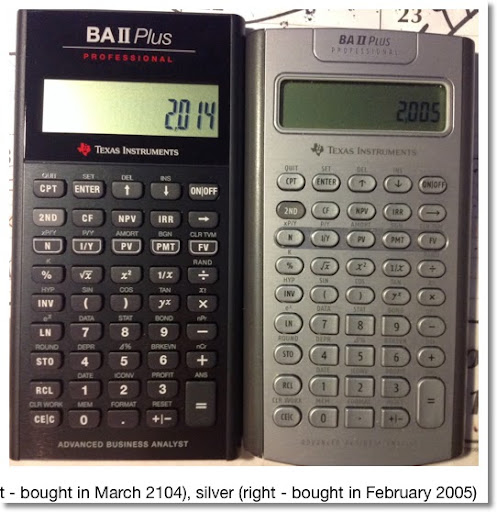First of all, I have to give a shoutout to Office Depot, who matched the price they had for the BA II Plus Professional on their website (\$49.99) instead of the store price (\$57.99).

Today's blog entry is about the BA II Plus Professional which is the deluxe version of the long time running BA II Plus. Like the "base" model, the BA II Plus Professional operates the same way: other than the standard Time Value of Money worksheet (TVM), you scroll through the options and calculations through each worksheet by using the up and down arrows.

What does the BA II Plus Professional have above the BA II Plus?

* The number of cash flows that can be entered is increased from 24 to 32
* The cash flow worksheet has additional calculations: net future value, payback, discounted payback.
* Modified internal rate of return is added.
* Modified duration of bonds is added.

Both have (to name a few):
* Trigonometric functions
* Chain or AOS mode (post-fix)
* Depreciation: Straight Line, Sum of Years' Digits, Declining Balance, Declining Balance with Crossover (good for taxes)
* Four Regressions for Statistics: Linear, Logarithmic, Exponential, Power (standard)
* Breakeven, Date Calculations (until 2049... ugh).
* Interest Conversion, Percent Change, and Amortization

Truthfully, both models have plenty to offer.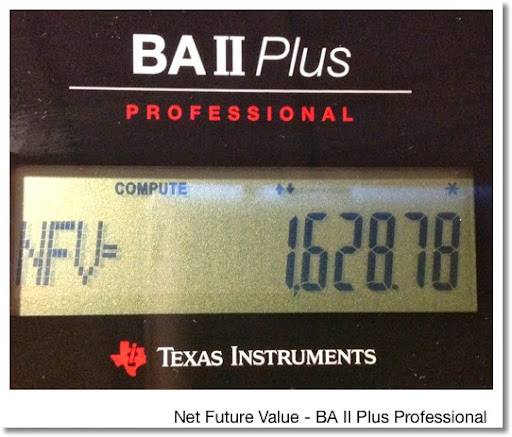Let's talk about keyboards The keyboard on the silver BA II Plus Professional is awful, at least on the one I have. The buttons are very hard to press, and even with hard presses keys do not register. At various angles the 2nd functions, which are light gray fade perfectly into the silver plate, making them not invisible.

The current black keyboard is miles ahead of the original keyboard. First of all. The printing of the 2nd functions, which are now in medium gray, are visible against the black plate. Second, the keys respond well and you don't need hammers as fingers to get the keys to register.

Displays are different. The black keyboard has a larger display. You can see the difference below.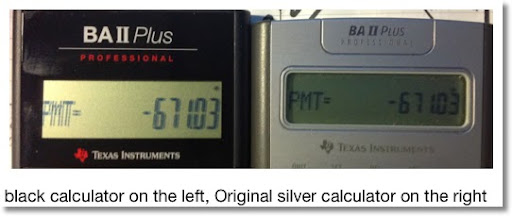Do I recommend the BA II Plus or the BA II Plus Professional? Truthfully it depends on needs (or wants) since there is a price difference between the two. Should you decide to get the BA II Plus Professional, I highly recommend that you get try current black keyboard.

By the way, Texas Instruments also redesigned the BA II Plus. The current keyboard is slight shorter and the keys are slightly bunched together. However, like the new BA II Professional, the display is bigger.

Let me know what you think in the comments. Maybe I should do a comparison between BA II Plus Professional vs HP 10bII+ or 12c Platinum? As always, thank you for your support and see you next time!

Eddie

This blog is property of Edward Shore. 2014

## Saturday, March 22, 2014

### Random Calculator Thoughts: New Design of the BA II Plus, Names of Calculators, Calculator Apps allowed on Tests in Texas

Texas Instruments:  New Design of the BA II Plus and BA II Plus Professional

Recently, Texas Instruments released a new design of their financial calculators, the BA II Plus (http://education.ti.com/en/us/products/calculators/financial-calculators/baii-plus/features/features-summary) and the BA II Plus Professional (http://education.ti.com/en/us/products/calculators/financial-calculators/baii-plus-professional/features/features-summary).

*  The display of the new BA II Plus and the Professional show larger digits and time-value-of-money indicators.
*  I first purchased a BA II Plus Professional when it was first released in 2004.  The original had a silver, metallic design with silver keys.  The keys on the one I had were really hard to press, making the calculator incredibly hard to use.   The keyboard certainly did not allow for long periods of use.  I am really hoping the new black design has a much better keyboard.
*  At first glance, the black design of the BA II Plus professional looked like a calculator that was specialized for the auto mechanic, not for the finance professional.  It still looks good, and better than the original silver design in my opinion.
* I don't own either calculators in the new design, but I plan to get the BA II Professional when I can.  If you do, please leave a comment.

The Names of Calculator Series

* Texas Instruments and Casio both like to keep the model numbers of their calculators the same.  This is both good and bad.  The good is that keeping the same number shows that the calculator, even with the new features, keeps the same lineage so going to the new version is easier.  The bad is that if there are so many versions, it is hard to tell which one to shop for, either online or in the store.  Bottom line, research and note the exact model name that you want.
*  Here are a few examples of such calculator lines:

TI-30 (Texas Instruments)

The Original TI-30 was released in 1976.  Jumping to today:

TI-30xa: basic battery powered model with the old-school post-fix entry

TI-30x Solar, known as the TI-30 ECO RS outside of the United States:  the solar version of the TI-30xa.   Unfortunately, Texas Instruments stopped selling the 30x Solar model in the United States.

TI-30 X II:  Two line calculator - now comes in a rainbow of colors.  I have a light blue one I got from Target several years ago.  The TI-30 XII gets linear regression and five storage registers.  Want a battery powered 30XII?  Shop outside the United States.

TI-30 X Multiview: Over the 30 XII, the Multiview gets the ability to input equations in textbook fashion and a function table.

TI-30X Pro/TI-36X Pro:  Both are the same.  In the United States, this is called the TI-36X Pro.  Outside the USA, it is called the TI 30X-Pro.  This is the top of the line in this line and the 36X Pro is one of my favorite calculators of all time.  Over the 30XII Multiview, we get calculus functions (integrals, derivatives, summations), an equation solver, Boolean algebra, matrices, vectors, and conversions.

TI-83/84 (Texas Instruments - their flagship graphing calculator)

TI-83 - without apps
TI-83 Plus
TI-83 Plus Silver Edition
TI-84 Plus
TI-84 Plus Silver Edition
TI-84 Plus C Silver Edition (this is the color graphing calculator)

Casio fx-115/fx-991 Series

Depending on where you live, these calculators are either called the fx-115 or the fx-991.  Several years back I did a review of the fx-115 ES Plus calculator where I talk about the newer features such of integer division, GCD, and LCM.  It turns out that that the fx-991 ES Plus did not have the new features I mentioned, which was brought up in the comments (thanks! :) ).  The international equivalent of the fx-115 ES Plus is the fx-991 EX Plus C, though I am not sure if the fx-991 ES Plus C is even available anymore.  Although the equivlaent for Japan is the fx-995 ES.

The fx-991 ES Plus which is still on sale according to the World Wide Casio website, by looking at the keyboard, the fx-991 ES Plus only has one more thing than the fx-115 ES, a random integer function.

Casio fx-3650p and fx-50f (Plus)

The fx-3650p and fx-50f are solar programmable calculators.  The fx-50f has the added bonus an equation library.    Despite the fact that the programming language on both of these calculators are simplified, it adds a powerful dimension.  It it also encourages students to learn programming to solve various mathematical problems.

Why are the fx-3650p and fx-50f not sold in the United States?  Drives me nuts!  I have a fx-3650p which ordered from a store in Hong Kong several years.

Here are links to the Casio world-wide site:

fx-50f Plus: http://edu.casio.com/products/program/fx50fp/

Apparently, there are now several varieties of the fx-50f.

Calculator Apps in the Classroom

I believe it is inevitable that calculator apps will become part of the norm in the classroom.  For comparison purposes, fifteen to twenty years ago, a lot of teachers shivered at the thought of allowing graphing calculators into the classroom.   Today, go to any math classroom (middle school, high school, or college), and the majority of the students will have a graphing calculator. Please correct me if I am wrong, but I think that the standardized tests of high school students have parts that require graphing calculators.

The reason why I am coming to the conclusion is because of an article I recently read.  Texas Commissioner of Education Michael Williams has decided to allow districts to use either a handheld graphing calculator or a graphing calculator app for the 8th grade mathematics portion of the STAAR (State of Texas Assessment of Academic Readiness).  The article states that it is the first time a graphing calculator app is allowed on a standardized test.  This a pilot program that will be used for the 2014-2015 academic school year.  I expect that graphing calculator apps will be fully accepted after the pilot program.  Note that smart phones are not still allowed.

Wow... 8th graders have smart phones - shows how old I am.

Here is a link to the article from the KLTV news site, one of the various news sources reporting on this:  http://www.kltv.com/story/25031254/tea-authorizes-use-of-calculator-app-for-14-15-staar-math-test.

That wraps up this blog entry - please let me your thoughts and comments.  As always they are much appreciated.  I'll talk to you next time!

Eddie

This blog is property of Edward Shore.  2014

## Sunday, March 16, 2014

### HP 12C: Value of a Deferred Monthly Annuity

HP 12C: Value of a Deferred Monthly Annuity

This is a continuation of sorts of the pension blog entry posted yesterday. Click on this link to read the blog entry regarding pensions.

Input - Store before running:
R0 = months to retirement
R1 = months of retirement (annuity)
PMT = monthly annuity payment
i = discount rate

Output:
1. Press R/S to get the value of all annuity
2. Press R/S to get the present value of the deferred annuity

Program:
Key: Step - Key Code
0: 01- 0
FV: 02 - 15
RCL 1: 03 - 45, 1
n: 04 - 11
PV: 05 - 13
R/S: 06 - 31
CHS: 07 - 16
FV: 08 - 15
0: 09 - 0
PMT: 10 - 14
RCL 0: 11 - 45, 0
n: 12 - 11
PV: 13 - 13
GTO 00: 14 - 43, 33, 00

Note: This is done on a "classic" HP 12C. With a HP 12C Platinum, use RPN mode and replace GTO 00 with GTO 000.

Examples:
An annuity that will pay \$2,000 a month for 324 months (27 years) will be paid 312 months (26 years) from now. Assume an annual discount rate of 5.5% (periodic rate of about 0.4583%).

Steps:
324 STO 0
312 STO 1
5.5 g 12÷
3000 PMT
R/S: Result: -505,785.53
R/S: Result: -121,435.08

The annuity needs to be funded with \$121,435.08 in order to meet the required payment structures 26 years from now.

Eddie

This blog is property of Edward Shore. 2014

## Saturday, March 15, 2014

### Finance: Approximating the Value of Pensions and Required Contributions

Greetings everyone!

Last week I was at a finance conference in Costa Mesa, CA for my work. It is a general accounting and tax conference that is held by my employer every year and topics covered range from sales tax, year-end reporting, using Microsoft Office to streamline processes, and the speech of why the university system I work for is important.

Unlike most attendees, I was invited last hour. 4:00 on the Friday before the conference. Despite having to reserve a room at the hotel across the street who at first mistakenly put my reservation for one month later, I had a great time.

One of the workshops I went to was about accounting for pensions (an area I usually don't work with at my job, it is good to step out of the comfort zone). There are two accounting regulations GASB 67 & 68 that were put into place in 2012. GASB is short for Governmental Standards Accounting Board. Both deal with how government entities valued pensions, determine pension liability, and make footnotes about them in the financial statements. I will include a link for reference for those who are interested:

GASB 67

GASB 68

Again, I don't claim to be an expert with either GASB 67 or 68, as my job usually doesn't involve working with pensions.

This workshop lead me to try to get an understanding of how pensions are valued. The examples I present on this blog entry is for one employee, not for an entire system of employers. Hopefully this will provide some sort of an introduction to anyone interested in pensions.

Types of Pensions

To my understanding, there are two general types of pensions:

Defined Benefit Plan: This plan details a promised payment (pension benefit) to an employee when the employee retires. The amount itself is determined in various ways, such as a percentage of a salary.

Defined Contributor Plan: The pension that is needed for the employee is funded by payments that contributed by the employer while the employee is working.

It would not surprise me if there are pension plans that have characteristics of both a defined benefit and defined contributor plans.

Valuing Pensions

In simple terms, pensions can be thought of a "deferred annuities". Pensions have two parts to them:

* Before retirement, where the employee works. Pensions can be funded by a one-time deposit or by monthly contributions. My pension is the latter.

* After retirement, where the employee receives the pension benefits. The length of time when the employee receives benefits is determined by professional actuaries.

I am going to use my trusty HP 12C calculator for these examples, but any financial calculator will do. Again, the examples I list here are simplified.

Example 1

Situation and Assumptions:
* An employee is currently 42 years of age and wants to retire at the age of 67.
* An actuary determines that the employee will live to the age of 90. Hence, the length of pension benefits will be for 23 years. Assume that this is going to be pretty certain (see note below).

In reality, actuary use probability factors that an employee lives to certain age. Higher ages of mortality receive less probability than younger ages. I think the standard highest age anyone is considered to live is 110 years.

Often determining the value of present value will involve calculating a factor, determine by actuaries, to be used in the formula:

PV of Pension Benefits Paid after Retirement = Payment × Pension Factor

In our example, we will assume each payment will occur with 100% certainty.

* The discount rate is 5% compounded annually. (The periodic rate would be about 0.4167%)
* The monthly payment is determined to be \$3,000 paid at the end of the month. Pensions benefits can also be paid at the beginning of the month as well.
* The employer plans to make a one-time deposit in account that accrues a safe 5% interest rate.

What is the current value of the pension (when the employee is at age 42)?

We divide this problem into two calculations:
1. Present Value of the pension benefits paid from age 67 to age 90.
2. Present Value of the "lump sum" discounted back to age 42 to determine the one-time required contribution, when the employee turns 42.

Part 1:
n = 23 × 12 = 276
i = 5 ÷ 12 = 0.4167 (I/YR = 5, P/PY = 12)
PMT = 3,000.00
FV = 0.00
Calculated PV = -491,476.19 This amount becomes the FV in Part 2. I enter this amount as a positive in Part 2.

Part 2:
n = 25 × 12 = 300
i = 5 ÷ 12 = 0.4167 (I/YR = 5, P/PY = 12)
PMT = 0.00
FV = 491,476.19
Calculated PV = -141,176.44

The employer will have to deposit \$141,176.44 into an account paying 5% interest. It is the current value of this pension.

Example 2

Situation and Assumptions:
* An employee is currently 37 years of age and wants to retire at the age of 67.
* An actuary determines that the employee will live to the age of 92. Hence, the length of pension benefits will be for 25 years.
* The discount rate is 5% compounded annually. (The periodic rate would be about 0.4167%)
* The monthly payment is determined to be \$3,000 paid at the end of the month.
* The employer plans to make monthly contributions to the pension. We assume that the employee will be working for the entire 30 years.

What is the required monthly payment while the employee is working?

We divide this problem into two calculations:
1. Present Value of the pension benefits paid from age 67 to age 92.
2. Present Value of the "lump sum" discounted back to age 37 to determine the monthly contributions.

Part 1:
n = 25 × 12 = 300
i = 5 ÷ 12 = 0.4167 (I/YR = 5, P/PY = 12)
PMT = 3,000.00
FV = 0.00
Calculated PV = -513,180.14 This amount becomes the FV in Part 2. I enter this amount as a positive in Part 2.

Part 2:
n = 30 × 12 = 300
i = 5 ÷ 12 = 0.4167 (I/YR = 5, P/PY = 12)
PV = 0.00
FV = 513,180.14
Calculated PMT = -616.61

At the end of each month, the employer contributes \$616.61 to the pension.

That is my introduction to pensions, hope you find this helpful.

Eddie

Resources (retrieved during 3/10/14 - 3/15/14)

Determining the Present Value of a Pension Benefit - FinanceMom

Pension Value of a Defined Benefit Pension - Pension Analysis Consultants, Inc. See section 16.13

This blog is property of Edward Shore. 2014

## Friday, March 14, 2014

### Happy π Day! Happy Birthday Albert Einstein!Today is a great day. We get to celebrate one of the best numbers in existence: π. π plays a role in many mathematical and scientific applications. Some examples include:

Area of a Circle = π * radius^2

Area of an Ellipse = π * length of semi-major axis * length of semi-minor axis

The functions sin x, cos x, and tan x each have a period of π.

Euler's Formula: e^(i*π) + 1 = 0

Definite integral involving π:
∫(e^(-a*x^2) dx, 0, infinity) = 0.5 * √(π/a)

1 + 1/3 + 1/5 + 1/7 + ... = sum of the fractions of 1/odd integers = π/4
(Convergence might be slow)

Γ(1/2) = √π. (Gamma of 1/2)

Probability: Area under the Normal Curve:
1/√(2 π) * ∫(e^(-x^2/2))

Angular Velocity, general: ω = 2 π f, where f is frequency

Angle in Radians = Angle in Degrees * π/180

Optics: Double Split Interference - Intensity at an Angle:
I = 4 * I_0 * cos( π * d * sin θ / λ )^2

Also today is Albert Einstein's birthday (March 14). He is primarily responsible for the Theory of Relativity and E = mc^2. I am going to give myself a refresher course.

Here is some information about the Theory of Relativity:
http://en.wikipedia.org/wiki/Theory_of_relativity

This blog is property of Edward Shore. 2014

## Saturday, March 1, 2014

### HP Prime/TI 84 Plus Color Silver Edition: Dynamic Programming: Orbit on a elliptic path.

This program orbits a θ character on a elliptical path. Use the arrow keys to change the size and the elliptical path. The program executes until ENTER is pressed.

Controls:
Left: Decrease of horizontal semi-axis
Right: Increase of horizontal semi-axis
Up: Increase of vertical semi-axis
Down: Decrease of vertical semi-axis
ENTER: Exits the program

HP Prime
EXPORT ORBIT( )
BEGIN
LOCAL A,B,K,I,X,Y;

// Initialize
A:=25; B:=25; I:=0;
X:=160+A*COS(I);
Y:=120-B*SIN(I);
MSGBOX("Press Enter to exit.");
RECT( );

REPEAT
// clear old placements and text
RECT_P(X,Y,X+10,Y+12);
RECT_P(0,218,319,239);

// get input from keyboard
K:=GETKEY;

IF K==7 AND A>1 THEN
A:=A+1; END;
IF K==8 AND A<50 THEN
A:=A-1; END;
IF K==2 AND B<50 THEN
B:=B+1; END;
IF K==12 AND B>1 THEN
B:=B-1; END;

// Move the satellite
I:=I+π/64;
X:=160+A*COS(I);
Y:=120-B*SIN(I);
TEXTOUT_P("θ",X,Y,2);
TEXTOUT_P("A = "+STRING(A)+", B = "+STRING(B),
0,2,#FFh);
WAIT(0.05);
UNTIL K==30;
END;

TI-84 Color Plus Silver Edition
Note: Pixels on the newer Color edition (size 250 × 150 approximately for the graph screen) opposed to the classic black and white TI-84+ (62 × 94)

Program: ORBIT
: ZStandard
: ZSquare
: FnOff
: 5 → A
: 5 → B
: 0 → I
: ClrDraw
: AxesOff
: Repeat K=105
: Pt-Off(A cos(I), B sin(I), 2
: Text(150,0," " // 32 spaces - there is no box command
: GETKEY→ K
: If K=24 and A>1
: A-1 → A
: If K=26 and A<10
: A+1→ A
: If K=25 and B<10
: B+1→ B
: If K=34 and B>1
: B-1 → B
: I + π/16 → I
: Pt-On(A cos(I), B sin(I), 2
: Text(150,0,"A =",A,",B =",B
: End
: AxesOn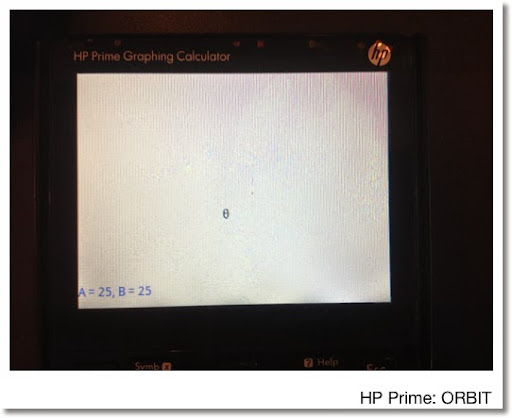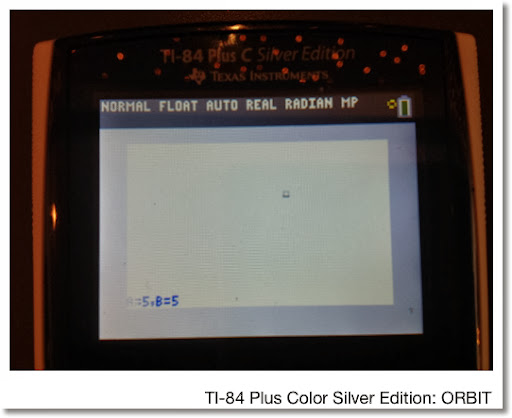This blog is property of Edward Shore. 2014

### TI-Nspire: Templates to Plot Functions, Parametric Equations, and Sequences

TI-Nspire:  Templates to Plot Functions, Parametric Equations, and Sequences Introduction The following are sample templates to plot functi...